# Blog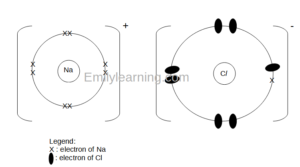## Drawing Dot-and-Cross Diagrams of Ionic Compounds – O Level Chemistry

In this post, let’s look at examples of dot-and-cross diagrams of ionic compounds for O Level Chemistry. Specifically, we will only be drawing the dot-and-cross…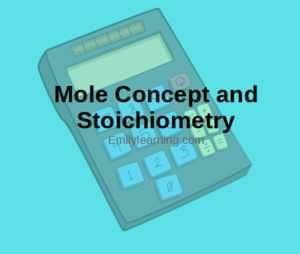## Mole Concept and Stoichiometry – Formulae for O Level Chemistry

In this post, you will find a summary of all the mole concept and stoichiometry formulae you’ll need for your O Level Chemistry exams.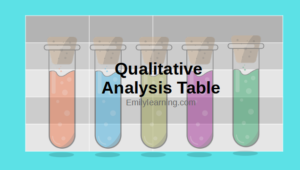## Qualitative Analysis Table – O Level Chemistry

In O Level Chemistry, students learn the qualitative analysis table of cations, anions and also testing for gases. Let’s look at these three groups of…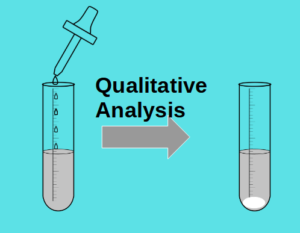## Qualitative Analysis – O Level Chemistry

Qualitative analysis is the process of identifying chemical components present in a sample by doing tests. Learn to write our observations in this post.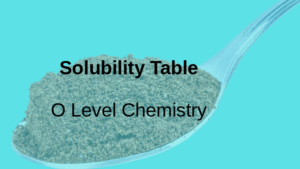## Solubility Table of common ionic compounds in water – O Level Chemistry

In this post, I’ll share the solubility table of common ionic compounds in water. Solubility table of common ionic compounds in water Below is the…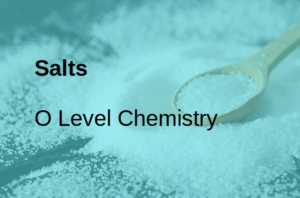## Salts – O Level Chemistry

In this post, let’s talk about salts as tested in O Level Chemistry. Check out our other posts on acids and bases which are very…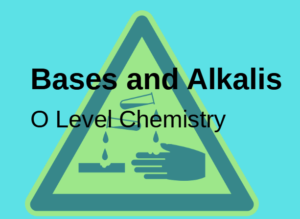## Bases and alkalis for O Level Chemistry

In this post, let’s look at bases and alkalis as tested in O Level Chemistry. What are bases bases and alkalis common properties of alkalis…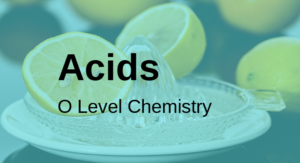## Acids for O Level Chemistry

In this post, let’s talk about acids for O Level Chemistry. What are acids Properties of acids Common acids Weak vs strong acids Acid reactions…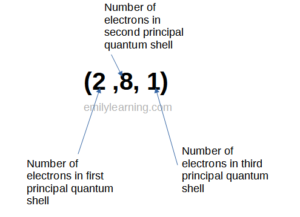## Write Electronic Configuration of atoms and ions – for O Level Chemistry

In this post, let’s look at how to write electronic configuration of atoms and ions the O Level Chemistry way. Here are the sections we…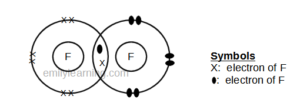## Drawing dot- and- cross diagrams of Covalent Molecules – O Level

In this post, let’s talk about drawing dot- and- cross diagrams of covalent molecules. A covalent bond is a chemical bond formed by sharing of…